# Lathe

95% of the components manufactured on the lathe comply with the standard, of which 80% of the components are first-class. How likely can we expect a manufactured part to be first class?

p =  76 %

### Step-by-step explanation:Did you find an error or inaccuracy? Feel free to write us. Thank you!Tips to related online calculators
Would you like to compute count of combinations?

## Related math problems and questions:

• Component fail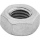There is a 90 percent chance that a particular type of component will perform adequately under high temperature conditions. If the device involved has four such components, determine the probability that the device is inoperable because exactly one of the
• Alarm systems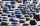What is the probability that at least one alarm system will signal the theft of a motor vehicle when the efficiency of the first system is 90% and of the independent second system 80%?
• Geography testsOn three 150-point geography tests, you earned grades of 88%, 94%, and 90%. The final test is worth 250 points. What percent do you need on the final to earn 93% of the total points on all tests?
• MasteringThe student masters the subject matter for the exam in Czech to 98%, from Math to 86% and from Economics to 71%. What is the probability that he will fail from Math and from others will succeed?
• Two doctorsDoctor A will determine the correct diagnosis with a probability of 93% and doctor B with a probability of 79%. Calculate the probability of proper diagnosis if both doctors diagnose the patient.
• Std-deviationCalculate standard deviation for file: 63,65,68,69,69,72,75,76,77,79,79,80,82,83,84,88,90
• The manufacturer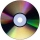The manufacturer found that 3% of the plates produced had a malfunction. Of the compliant, 75% are first and 25% second. What is the probability of producing first and second class plates?
• Dice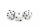How many times must throw the dice, the probability of throwing at least one six was greater than 90%?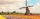How large must the group of people be so that the probability that two people have a birthday on the same day of the year is greater than 90%?
• Final examThere are 5 learners in a class that has written a final exam Aleta scored 55% vera scored 36% and Sibusiso scored 88% if Thoko scored 71% and the class average was 63%. What was Davids score as a percentage?
• Class - boys and girls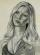In the class are 60% boys and 40% girls. Long hair has 10% boys and 80% girls. a) What is the probability that a randomly chosen person has long hair? b) The selected person has long hair. What is the probability that it is a girl?
• Doctors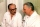The drug successfully treats 90% of cases. Calculate the probability that he will cure at least 18 patients out of 20?
• The vaccination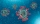The vaccination coverage of the population is 80%. Unvaccinated make up 60% of all infected. What percentage are unvaccinated more likely to be infected?
• The city 2Today lives 298000 citizens in the city. How many citizens can we expect in 8 years if their annual increase is 2.4%?
• Test scoresJo's test scores on the first four 100 point exams are as follows: 96,90,76, and 88. If all exams are worth the same percent, what is the minimum test score necessary on his last exam to earn an A grade in the class (90% or better)?
• Three sigma rule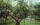The height of trees in a given stand is known to be a quantity with a normal probability distribution with a mean value of 15 m and a variance of 5 m2. Determine the interval in which there will be tree heights in such a stand with a probability of 90%
• Class enrollmentA grade 6 class went on a field trip only 98% or 49 pupils joined the activity. What was the class enrollment?